×#### Thank you for registering.

One of our academic counsellors will contact you within 1 working day.

Click to Chat

1800-1023-196

+91-120-4616500

CART 0

• 0

MY CART (5)

Use Coupon: CART20 and get 20% off on all online Study Material

ITEM
DETAILS
MRP
DISCOUNT
FINAL PRICE
Total Price: Rs.

There are no items in this cart.
Continue Shopping```Interval in Which the Roots LieIn some problems we want the roots of the equation ax2 + bx + c = 0 to lie in a given interval. For this we impose conditions on a, b, and c. Let f(x) = ax2 + bx + c.

(i)     If both the roots are positive i.e. they lie in (0, ¥), then the sum of the roots as well as the product of the roots must be positive.

Þa + b = –> 0 and ab =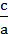> 0 with b2 – 4ac > 0.

Similarly, if both the roots are negative i.e. they lie in (–¥, 0) then the sum of the roots will be negative and the product of the roots must be positive.

i.e. a + b =< 0 and ab => 0 with b2 – 4ac > 0.

(ii)    Both the roots are greater than a given number k if the following three conditions are satisfied D > 0,> k and a.f(k) > 0.

(iii)    Both the roots will be less than a given number k if the following conditions are satisfied: D > 0,< k and a.f(k) > 0.

(iv)   Both the roots will lie in the given interval (k1, k2) if the following conditions are satisfied D > 0, k1 << k2 and a.f(k1) > 0,
a.f(k2) > 0.

(v)    Exactly one of the roots lies in the given interval (k1, k2) if f(k1). f(k2) < 0.

(vi)   A given number k will lie between the roots if a.f.(k) < 0.

In particular, the roots of the equation will be of opposite signs if 0 lies between the roots Þ a.f(0) < 0.

Illustration:

Find the values of the parameter a for which the roots of the quadratic equation x2 + 2(a – 1)x + a + 5 = 0 are

(i)     real and distinct,

(ii)    equal,

(iii)    not real,

(iv)   opposite in sign,

(v)    equal in magnitude but opposite in sign

(vi)   positive,

(vii)   negative,

(viii)  such that one root is greater than 3, and the other is smaller     than 3,

(ix)   greater than 3,

(x)    smaller than 3,

(xi)   such that exactly one root lies in the interval (1, 3),

(xii)   such that both the roots lie in the interval (1, 3),

(xiii)  such that one root is greater than 3 and the other root is smaller        than 1.

Solution:

Let f(x) = x2 + 2(a – 1)x + a + 5 = Ax2 + Bx + C    (say)

Þ A = 1, B = 2(a – 1), C = a + 5.

Also   D = B2 – 4AC = 4(a–1)2 –4(a + 5) = 4(a + 1)(a – 4).

(i)     D > 0 Þ (a + 1)(a–4) > 0

Þ a Î (–¥, –1)È(4, ¥)

(ii)    D = 0 Þ (a + 1)(a – 4) = 0 Þ a = –1, 4.

(iii)    D < 0 Þ (a + 1)(a – 4) < 0

Þ a Î (–1, 4).

(iv)   This means that 0 lies between the roots of the given equation.

Þ f(0) < 0 and D > 0 i.e. a Î (–¥, –1)È(4, ¥)

and a + 5 < 0 Þ a < –5

or a Î (–¥, –5).

(v)    This means that the sum of the roots is zero

Þ –2(a – 1) = 0 and D > 0 i.e. a Î (–¥, –1)È(4, ¥)

and a = 1

which does not belong to (–¥, –1)È(4, ¥) Þ a is null set f.

(vi)   This implies that both the roots are greater than zero

Þ –B/A > 0, C/A > 0, D > 0

Þ –(a – 1) > 0, a + 5 > 0, a Î (–¥, –1]È[4, ¥)

Þ a < 1, –5 < a, a Î (–¥, –1]È[4, ¥) Þ a Î (–5, –1].

(vii)   This implies that both the roots are less than zero

Þ –B/A < 0, C/A > 0, D > 0

Þ –(a – 1) < 0, a + 5 > 0, a Î (–¥, –1]È[4, ¥)

Þ a > 1, –5 > a, a Î (–¥, –1]È[4, ¥) Þ a Î [4, ¥).

(viii)  This means that 3 lies between the roots

Þ f(3) < 0 and D > 0

Þ 9 + 2(a – 1)(3) + a + 5 < 0 and a Î (–¥, –1)È(4,¥)

Þ a < –8/7 and a Î (–¥, –1)È(4, ¥)

Þ a Î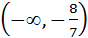.

(ix)   In this case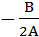> 3, A.f(3) > 0 and D > 0

Þ –(a – 1) > 3, 7a + 8 > 0 and a Î (–¥, –1]È[4, ¥)

Þ a < –2, a > –8/7 and a Î (–¥, –1]È[4, ¥).

Since no value of a can satisfy these conditions simultaneously, there can be no value of a for which both the roots will be greater than 3.

(x)    In this case> 3, A.f(3) > 0 and D > 0

Þ a < –2, a > –8/7 and a Î (–¥, –1]È[4, ¥)

Þ a Î(xi)   In this case f(1).f(3) < 0 and D > 0

Þ (3a + 4)(7a + 8) < 0 and a Î (–¥, –1]È[4, ¥)

Þ a Î.

(xii)   In this case 1 << 3, A.f(1) > 0, A.f(3) > 0, D > 0.

Þ 1 < –1(a – 1) < 3, 3a + 4 > 0, 7a + 8 > 0, aÎ(–¥,–1]È[4,¥)

Þ a Î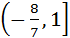.

(xiii)  In this case D > 0 and f(1) < 0 i.e. 3a + 4 < 0

Þ a < –4/3

and f(3) < 0 i.e. 7a + 8 < 0

Þ a < –8/7 and a Î (–¥, –1)È(4, ¥).

Combining we get, a Î (–¥, –4/3).

Illustration:

If a is a root of the equation ax2 + bx + c = 0 and b is a root of the equation –ax2 + bx + c = 0, then prove that there will be a root of the equationx2 + bx + c = 0 lying between a and b.

Solution:

Let f(x) =x2 + bx + c = 0

Þ f(a) =a2 + ba + c = aa2 + ba + ca2

=a2                    (As a is a root of ax2 + bx + c = 0)

Also f(b) =b2 + bb + c = –ab2 + bb + c +ab2.

=ab2 (As b is a root of – ax2 + bx + c = 0).

Now f(a).f(b) =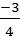a2a2b2 < 0 Þ f(x) = 0 has one real root between a and b.

Illustration:

Find the values of ‘a’ for which 4t – (a – 4)2t + 9/4a < 0t Î (1, 2).

Solution:

Let 2t = x and f(x) = x2 – (a – 4) x + 9/4a.

We want f(x) < 0x Î (21, 22) i.e.x Î (2, 4)

(i)     Since coefficient of x2 in f(x) is positive, f(x) < 0 for some x only when roots of f(x) = 0 are real and distinct.

Þ D > 0 Þ a2 – 17a + 16 > 0 or a > 16.                        …… (1)

(ii)    Since we want (f)x < 0x Î (2, 4), one of the roots of f(x) should be less than 2 and the other must be greater than 4 i.e. f(2) < 0 and f(4) < 0. a < –48 and a > 128/7, which is not possible.

Hence no such ‘a’ exist.

To read more, Buy study materials of Quadratic Equation comprising study notes, revision notes, video lectures, previous year solved questions etc. Also browse for more study materials on Mathematics here.
```### Course Features

• 731 Video Lectures
• Revision Notes
• Previous Year Papers
• Mind Map
• Study Planner
• NCERT Solutions
• Discussion Forum
• Test paper with Video Solution